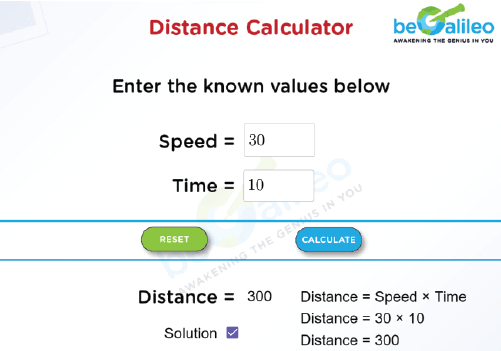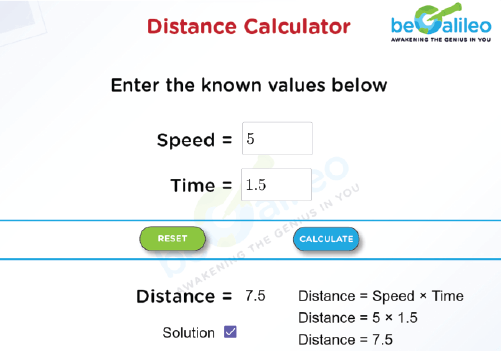>### Master Math with 1:1 Online Classes. Learn from Experts!## Distance Calculator

A distance calculator is a tool that simplifies the task of finding out how far you can travel based on two key pieces of information: your speed and the time it takes to reach your destination. This uncomplicated yet valuable tool has applications in various aspects of life, from arranging road trips to solving scientific problems. In our fast-paced world, mastering the use of a distance calculator, employing variables like speed and time, can be a significant advantage.

## How to use the Distance Calculator?

Here's how a distance calculator works step by step:

1. Input Variables :
Enter the variables for which you want to calculate the distance.
i.e., Speed (S): This is the rate at which the object is traveling, typically measured in miles per hour (mph), kilometers per hour (km/h), or meters per second (m/s). Time (T): This is the duration of the travel, usually measured in hours (h) or seconds (s).
2. Calculation :
The calculator multiplies the speed (S) by the time (T) according to the formula. This calculates the distance (D) covered during the specified time at the given speed.
3. View the Result :
The converted weight in kilograms will be displayed below the Converter. This is the equivalent weight in kg for the pounds you entered.
4. Output :
The result of the calculation is the distance traveled (D), usually expressed in the same unit as the speed used.
5. Reset Option :
If needed, you can use the reset button to clear all entered numbers, allowing you to correct input errors or start a new calculation.
If you want to view the calculation then click on the ‘Show Solution’ checkbox.

## How to find the Distance?

A distance calculator works by using a simple mathematical formula to calculate the distance traveled based on two key parameters: speed and time. The formula used is:

Distance (D) = Speed (S) × Time (T)

Example: You're traveling at a speed of 50 miles per hour for 2.5 hours. How far did you travel?

Solution : Speed (S) = 50 miles per hour

Time (T) = 2.5 hours

Apply the Distance Formula: Distance (D) = Speed (S)\timesTime (T)
= 50\times2.5
= 125 miles

Therefore, you traveled 125 miles.

## Solved Examples

### Q1. An object moves at a speed of 30 meters per second for 10 seconds. Find the distance traveled by the object.

Solution :
Using the distance formula, D = S\timesT :
D = 30 m/s\times10 seconds
D = 300 meters
Therefore, the object will travel a distance of 300 meters during this time.### Q2.You walk at a speed of 5 kilometers per hour for 1.5 hours. How far did you travel?

Solution :
Using the distance formula, D = S\timesT :
D = 5 km/h\times1.5 hours
D = 7.5 km
Therefore, you will cover a distance of 7.5 kilometers during your walk.